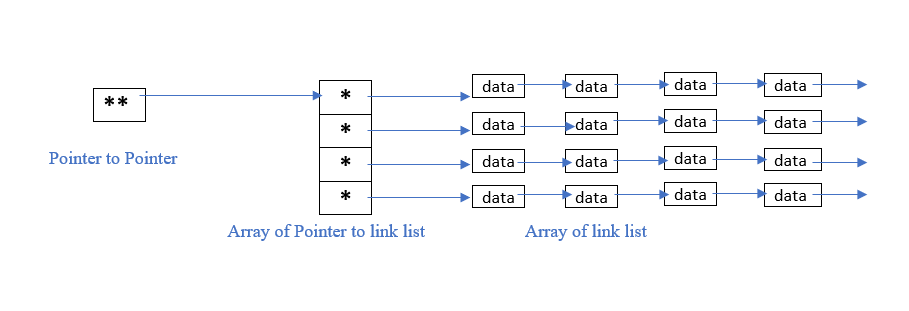# Array of Linked Lists in C/C++

• Difficulty Level : Easy
• Last Updated : 11 Oct, 2021

An array in C/C++ or be it in any programming language is a collection of similar data items stored at contiguous memory locations and elements can be accessed randomly using indices of an array.  They can be used to store the collection of primitive data types such as int, float, double, char, etc of any particular type. To add to it, an array in C/C++ can store derived data types such as structures, pointers, etc. Given below is the picture representation of an array.We provide nothing but the best curated videos and practice problems for our students. Check out the C Foundation Course and master the C language from basic to advanced level. Wait no more, start learning today!

A linked list is a linear data structure consisting of nodes where each node contains a reference to the next node. To create a link list we need a pointer that points to the first node of the list.

Approach: To create an array of linked lists below are the main requirements:

• An array of pointers.
• For keeping the track of the above-created array of pointers then another pointer is needed that points to the first pointer of the array. This pointer is called pointer to pointer.

Below is the pictorial representation of the array of linked lists:Below is the C++ program to implement the array of linked list:

## C++

 `// C++ program to illustrate the array``// of Linked Lists``#include ``using` `namespace` `std;`` ` `// Structure of Linked Lists``struct` `info {``    ``int` `data;``    ``info* next;``};`` ` `// Driver Code``int` `main()``{``    ``int` `size = 10;`` ` `    ``// Pointer To Pointer Array``    ``info** head;`` ` `    ``// Array of pointers to info struct``    ``// of size``    ``head = ``new` `info*[size];`` ` `    ``// Initialize pointer array to NULL``    ``for` `(``int` `i = 0; i < size; ++i) {``        ``*(head + i) = NULL;``    ``}`` ` `    ``// Traverse the pointer array``    ``for` `(``int` `i = 0; i < size; ++i) {`` ` `        ``// To track last node of the list``        ``info* prev = NULL;`` ` `        ``// Randomly taking 4 nodes in each``        ``// linked list``        ``int` `s = 4;`` ` `        ``while` `(s--) {`` ` `            ``// Create a new node``            ``info* n = ``new` `info;`` ` `            ``// Input the random data``            ``n->data = i * s;``            ``n->next = NULL;`` ` `            ``// If the node is first``            ``if` `(*(head + i) == NULL) {``                ``*(head + i) = n;``            ``}``            ``else` `{``                ``prev->next = n;``            ``}``            ``prev = n;``        ``}``    ``}`` ` `    ``// Print the array of linked list``    ``for` `(``int` `i = 0; i < size; ++i) {``        ``info* temp = *(head + i);`` ` `        ``// Linked list number``        ``cout << i << ``"-->\t"``;`` ` `        ``// Print the Linked List``        ``while` `(temp != NULL) {``            ``cout << temp->data << ``" "``;``            ``temp = temp->next;``        ``}`` ` `        ``cout << ``'\n'``;``    ``}`` ` `    ``return` `0;``}`
Output:
```0-->    0 0 0 0
1-->    3 2 1 0
2-->    6 4 2 0
3-->    9 6 3 0
4-->    12 8 4 0
5-->    15 10 5 0
6-->    18 12 6 0
7-->    21 14 7 0
8-->    24 16 8 0
9-->    27 18 9 0
```

My Personal Notes arrow_drop_up